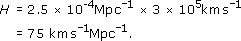Science, Maths & Technology

### Become an OU student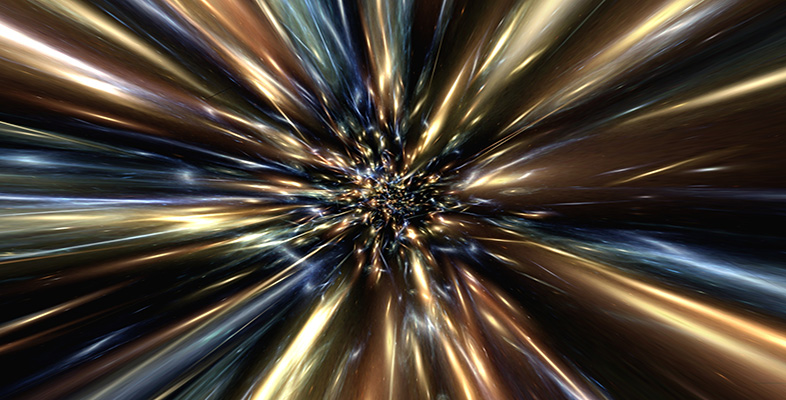The Big Bang

Start this free course now. Just create an account and sign in. Enrol and complete the course for a free statement of participation or digital badge if available.

# 4 The variation of redshift with distance

## 4.1 Hubble's discoveries

In this section, we bring together two important features of galaxies – their redshifts and their distances.

This crucial development owes its origins to Edwin Hubble. His pioneering work in 1923 first led to the confirmation that certain of the fuzzy patches in the sky, loosely called ‘nebulae’, were in fact galaxies like our own.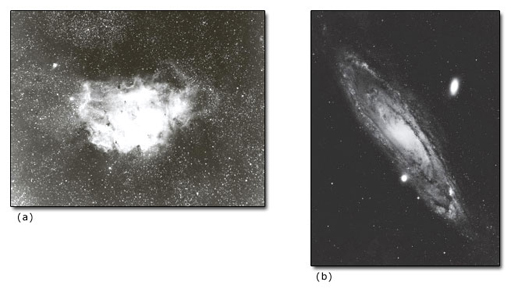(a) courtesy of Uppsala University; (b) Hale Observatories ©
(a) courtesy of Uppsala University; (b) Hale Observatories
Figure 14 (a) Nebula NGC 6514, a cloud of gas and dust in our own Galaxy; (b) Hubble showed that objects like M31 (NGC 224, now called the Andromeda galaxy) were galaxies like our own, and they ceased to be called nebulae. The two large bright patches near M31 are satellite galaxies, NGC 205 and NGC 221

It was immediately realised that the Universe was enormously bigger than had previously been thought. Also the number of galaxies was large. In fact, it is now known that the number of galaxies accessible to our telescopes is comparable with the number of stars in our Galaxy – about 100 billion (i.e. 1011).

A second significant discovery made by Hubble concerned the spectra of galaxies, nearly all of which are redshifted. This redshift was a systematic shift of all the lines to the red end of the spectrum (it was discovered by another US astronomer, Vesto Slipher). Using his measurements of distance, Hubble showed that the redshift increased with distance. As far as he could tell, the redshift of a galaxy was proportional to its distance.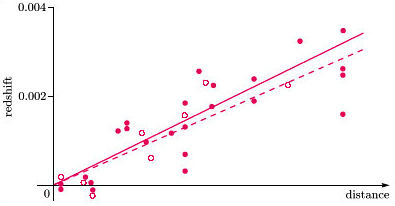based on E. P. Hubble (1929) in Proc. Nat. Acad. Sci., 15, 168 ©
based on E. P. Hubble (1929) in Proc. Nat. Acad. Sci., 15, 168
Figure 15 The redshift–distance relationship for galaxies, as plotted by Hubble in 1929. The solid line represents the relationship inferred from individual galaxies (solid circles), the dashed line the relationship when the galaxies are combined into groups (open circles). Hubble's distance scale has been omitted since it is now known that it was in error.

Hubble's original measurements, shown in Figure 15, exhibited a large scatter about a straight line. This was partly due to the inevitable observational uncertainties, especially in distance measurements. But even if one were able to remove the observational uncertainties, there would still have been a considerable scatter about a straight line. This comes from the fact that most of the galaxies Hubble looked at were members of clusters, and each was moving about within its cluster. Because the galaxies were rather close to us, the speeds associated with this random motion were comparable to the recessional speed Hubble was trying to measure. Sometimes the motion of the galaxy relative to the cluster centre was directed towards us (giving a blueshift component), sometimes away from us (adding a further redshift component). If the structure of the cluster were well enough known, these effects could, in principle, be estimated and a correction applied. Allowance must also be made for small gravitational redshifts (see Question 2). Finally, the motion of the Earth with respect to our Local Group must be subtracted. (This will be discussed in Section 6.2.4.) Suppose that all these corrections could be perfectly made in all cases. Then a redshift (z) against distance (r) plot would, as far as we know, appear as in Figure 16.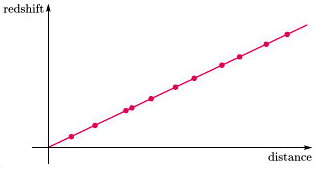Figure 16 An idealised Hubble diagram with all sources of scatter removed

The straight line in Figure 16 would represent the underlying cosmological redshift. Thus, for this cosmological redshift, we have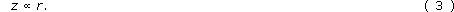Interpreting the observed redshift as a Doppler shift implies that each galaxy is receding at a speed proportional to its distance from us. To see this, consider the Doppler shift formula and the relationship between redshift, wavelength change and frequency shift given in the answer to Question 3. It follows that the redshift, z, is given by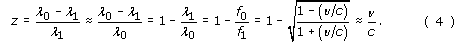Hence, Equations 3 and 4 together imply that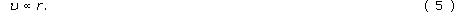This provides the basis for one of the common ways of writing Hubble's law,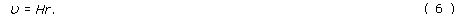The factor of proportionality, H, is sometimes called the Hubble constant. But the term parameter is perhaps preferable since the word ‘constant’ might lead one to think that it should remain constant in time – instead of being a constant of proportionality between two variables as those variables are at a particular point in time. H must, we now realise, vary slowly with time.

If the speed of recession is proportional to distance, this implies all distances between galaxies are increasing at the same rate. Not only do all clusters of galaxies appear to be receding from us here on Earth, they would appear to be receding in exactly the same manner from whatever vantage point an observer adopted.

Hubble's work has been continued, refined, and extended to much more distant and fainter galaxies.

### Question 6

Taking typical intercluster distances to be approximately 2 × 108 ly, and the value of H to be about 2 × 10−18 s−1, estimate the minimum z value that can reliably be ascribed to the expansion of the Universe. Compare this with the redshifts measured by Hubble in Figure 15.

To get a redshift that can confidently be regarded as due to expansion, we must look to another cluster.

Intercluster distances are approximately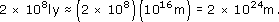The Hubble constant is about 2 × 10−18 s−1

Hence the cosmological redshift of a neighbouring cluster is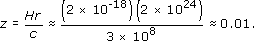Compare this with Figure 15 – Hubble was not able to look far enough!

Since it is hard to measure the distance to a far-off galaxy, it is not surprising that there has been a good deal of controversy about the reliability of distance estimates. The redshift measurements are much easier to make and are more direct, so there has been much less uncertainty over them. Nevertheless, it is useful to have a check, and this has been provided by radio astronomers measuring the redshift of lines in the radio spectrum, such as that due to the emission of hydrogen at a wavelength of 21 cm. This confirms that the redshift is the same over a large range of frequencies.

Based on a range of recent results, the value of H is currently reckoned to be 2.3 × 10−18 s−1, with an observational uncertainty of about 10%.

Hubble, who interpreted redshifts in terms of recessional velocities, would quote this as 23 km s−1 for every million light-years of distance of a galaxy. Estimates of H are often quoted in terms of km s−1 Mpc−1. In these units, H is about 70 km s−1 Mpc−1.

It has become common usage to write H = h × 100 km s−1 Mpc−1, in which case h currently has a best estimate of 0.7.

We end this section with a more up-to-date version of the Hubble diagram (Figure 17) for some galaxies and clusters of galaxies.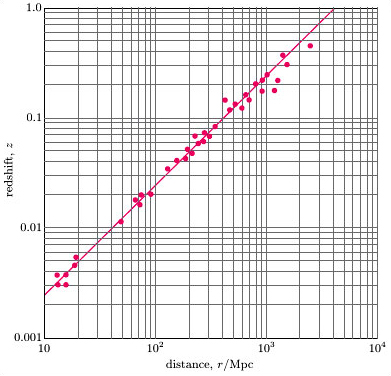adapted from A. Sandage (1970) Physics Today, vol. 34, February 1970, American Institute of Physics ©
adapted from A. Sandage (1970) Physics Today, vol. 34, February 1970, American Institute of Physics
Figure 17 A plot of redshift against distance for a selection of galaxies and clusters of galaxies

### Question 7

Use the sum of the shifts calculated for the star specified in Question 2 to give an estimate of the uncertainty in the cosmological redshift z for a star in a galaxy at 4 × 106 ly from us. (This may be an overestimate because the centre of a line can be estimated quite well even if the line is fuzzy. However, in a real galactic spectrum there will be a further component of broadening due to galactic rotation.)

From Question 2(b), parts (ii) and (iii), the minimum width of the lines is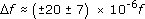with a systematic fractional shift of about −2 × 10−6 (Question 2(b), (i)). Thus the contributions to Δf/f due to turbulence, rotation and gravitational redshift vary between (+20 + 7 − 2) × 10−6 and (−20 – 7 – 2) × 10−6. This gives an error of about ±3 × 10−5. For a galaxy at 4 × 106 ly (≈4 × 106 × 1016 m), the expected cosmological redshift is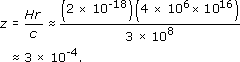Thus the line-broadening effects are approximately a fraction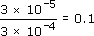of the expected cosmological shift.

Notice that a distance of 4 × 106 ly corresponds to nearby galaxies in our Local Group and the relative uncertainties in redshift will decrease with distance. They are commonly less than 1%. The uncertainties in distance, however, will increase with distance, because less accurate estimation procedures have to be used. Also note that a distance of 4 × 106 ly may be unreasonably small for cosmological consideration.

### Question 8

By taking appropriate readings off the graph of Figure 17, estimate the value of the Hubble constant, H, indicated by this set of data.

One can use any pair of points on the straight line graph that has been drawn, but to minimise reading errors we take the extreme points. The difference in z is 1.0000 − 0.0025 = 0.9975. The difference in distance, r, is 4000 − 10 = 3990 Mpc.

Thus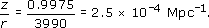But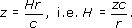So